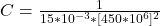## An MRI machine needs to detect signals that oscillate at very high frequencies. It does so with an LC circuit containing a 15mH coil. To wha

Question

An MRI machine needs to detect signals that oscillate at very high frequencies. It does so with an LC circuit containing a 15mH coil. To what value should the capacitance be set to detect a 450 MHz signal?

in progress 0
6 months 2021-08-15T15:20:21+00:00 1 Answers 34 views 0

## Answers ( )

The capacitance is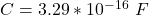Explanation:

From the question we are told that

The  induction of the LC circuit is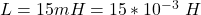The  frequency is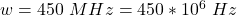The natural frequency is mathematically represented as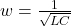Where C is the capacitance So

=>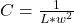substituting values